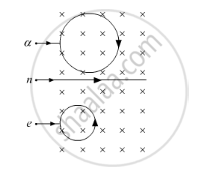# A Neutron, an Electron and an Alpha Particle, Moving with Equal Velocities, Enter a Uniform Magnetic Field Going into the Plane of the Paper, as Shown. Trace Their Paths in the Field and Justify Your Answer. - Physics

A neutron, an electron and an alpha particle, moving with equal velocities, enter a uniform magnetic field going into the plane of the paper, as shown. Trace their paths in the field and justify your answer.#### Solution

We know that a charged particle will experience a force when it enters a magnetic field. The magnetic field will move the charged particle in a circular path, as the force is perpendicular to the velocity of particle. The radius of the circular path will be given by

(mv^2)/r=Bqv

r=(mv)/(Bq)

As B and v are constant, we can write

rpropm/q

The neutron will move along the straight line as it has no charge.

The electron will inscribe a circle of radius smaller than that of the alpha particle as the mass to charge ratio of the alpha particle is more than that of the electron.

So, the alpha particle will move in the clockwise direction and the electron will move in anticlockwise direction according to the right-hand rule.Concept: Force on a Moving Charge in Uniform Magnetic and Electric Fields
Is there an error in this question or solution?